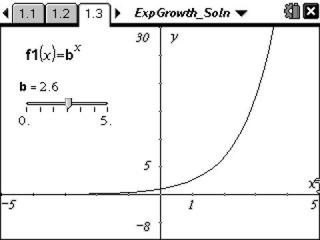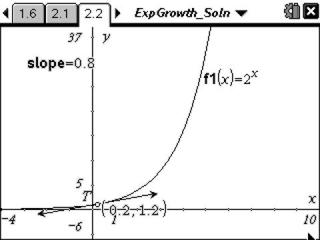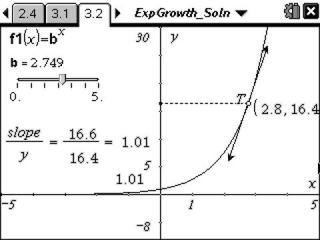# Activities

••• ##### Subject Area

• Math: Algebra II: Logarithms and Exponentials
• Math: College Algebra: Exponential and Logarithmic Functions

• ##### Author9-12

45 Minutes

• ##### Device
• TI-Nspire™ Navigator™
• TI-Nspire™
• TI-Nspire™ CAS
• ##### Software

TI-Nspire™
TI-Nspire™ CAS

2.0

• ##### Report an Issue

Exponential Growth#### Activity Overview

Students find an approximation for the value of the mathematical constant e and to apply it to exponential growth and decay problems.

#### Key Steps

•In Problem 1, students investigate the how the value of b affects the shape of the graph f(x) = bx.

•In Problem 2, students use a tangent line to the curve at a point to explore the relationship between the slope of the tangent line at that point and the value of the function at the same point.

•In the third problem, students combine the ideas of the graphs from the previous two problems. They observe the changing values to form conclusions about how the slope of the tangent line and the value of the function are related.